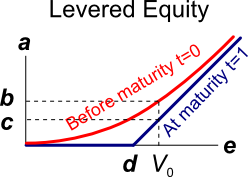# Fight Finance

#### CoursesTagsRandomAllRecentScores

The equations for Net Income (NI, also known as Earnings or Net Profit After Tax) and Cash Flow From Assets (CFFA, also known as Free Cash Flow to the Firm) per year are:

$$NI=(Rev-COGS-FC-Depr-IntExp).(1-t_c)$$

$$CFFA=NI+Depr-CapEx - \varDelta NWC+IntExp$$

For a firm with debt, what is the amount of the interest tax shield per year?

Which of the following discount rates should be the highest for a levered company? Ignore the costs of financial distress.

A risky firm will last for one period only (t=0 to 1), then it will be liquidated. So it's assets will be sold and the debt holders and equity holders will be paid out in that order. The firm has the following quantities:

$V$ = Market value of assets.

$E$ = Market value of (levered) equity.

$D$ = Market value of zero coupon bonds.

$F_1$ = Total face value of zero coupon bonds which is promised to be paid in one year.The levered equity graph above contains bold labels a to e. Which of the following statements about those labels is NOT correct?

A fairly priced unlevered firm plans to pay a dividend of $1 next year (t=1) which is expected to grow by 3% pa every year after that. The firm's required return on equity is 8% pa. The firm is thinking about reducing its future dividend payments by 10% so that it can use the extra cash to invest in more projects which are expected to return 8% pa, and have the same risk as the existing projects. Therefore, next year's dividend will be$0.90. No new equity or debt will be issued to fund the new projects, they'll all be funded by the cut in dividends.

What will be the stock's new annual capital return (proportional increase in price per year) if the change in payout policy goes ahead?

Assume that payout policy is irrelevant to firm value (so there's no signalling effects) and that all rates are effective annual rates.

The below screenshot of Commonwealth Bank of Australia's (CBA) details were taken from the Google Finance website on 7 Nov 2014. Some information has been deliberately blanked out.What was CBA's approximate payout ratio over the 2014 financial year?

Note that the firm's interim and final dividends were $1.83 and$2.18 respectively over the 2014 financial year.

The following cash flows are expected:

• Constant perpetual yearly payments of $70, with the first payment in 2.5 years from now (first payment at t=2.5). • A single payment of$600 in 3 years and 9 months (t=3.75) from now.

What is the NPV of the cash flows if the discount rate is 10% given as an effective annual rate?

In the dividend discount model (DDM), share prices fall when dividends are paid. Let the high price before the fall be called the peak, and the low price after the fall be called the trough.

$$P_0=\dfrac{C_1}{r-g}$$

Which of the following statements about the DDM is NOT correct?

A company has a 95% daily Value at Risk (VaR) of $1 million. The units of this VaR are in: The arithmetic average continuously compounded or log gross discrete return (AALGDR) on the ASX200 accumulation index over the 24 years from 31 Dec 1992 to 31 Dec 2016 is 9.49% pa. The arithmetic standard deviation (SDLGDR) is 16.92 percentage points pa. Assume that the log gross discrete returns are normally distributed and that the above estimates are true population statistics, not sample statistics, so there is no standard error in the sample mean or standard deviation estimates. Also assume that the standardised normal Z-statistic corresponding to a one-tail probability of 2.5% is exactly -1.96. If you had a$1 million fund that replicated the ASX200 accumulation index, in how many years would the mean dollar value of your fund first be expected to lie outside the 95% confidence interval forecast?

Suppose the market expects the Reserve Bank of Australia (RBA) to increase the policy rate by 25 basis points at their next meeting. The current exchange rate is 0.8 USD per AUD.

Then unexpectedly, the RBA announce that they will leave the policy rate unchanged due to increasing unemployment and fears of a potential recession.

What do you expect to happen to Australia's exchange rate on the day when the surprise announcement is made? The Australian dollar is likely to: